About   Products   Support   Videos   Purchase   Contact

 Free email newsletter with articles on systematic trading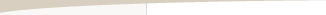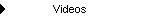Stress Testing for Trading Strategy Robustness

by Michael R. Bryant

In the article on multi-market trading strategies, I discussed the concept of robustness, which I described as insensitivity to variations in the data on which the strategy is based. Building a trading system over multiple markets is one way to increase robustness. However, what if you already have a strategy and you want to see how robust it is?

Testing a trading strategy for robustness is often referred to as sensitivity analysis, or more colloquially as stress testing. The basic idea is to see what happens when small changes are made to the strategy inputs, price data, or other elements of the strategy or the trading environment. A robust strategy exhibits a proportional and relatively muted reaction to such changes, whereas a strategy that is not robust will react disproportionally and sometimes fail outright when small changes are made to its inputs or environment.

Why is This Important?

Put simply, robustness is important because the markets never stay the same. Take the strategy inputs, for example. Inputs such as the look-back length for a moving average might be optimal over the back-test period, but going forward, different values might be optimal. We want to know how well the strategy will perform when the inputs are no longer optimal. One way to address that is to see how the results change when the input values are changed.

As explained in the earlier article, the idea of robustness is related to strategy over-fitting. We want to make sure that the strategy has not been fit so tightly to the market during the development process that it can't withstand any changes to the market. Generally speaking, we can test for that by changing the market, changing the strategy, or both. A strategy that does not stand up well to relatively small changes is not robust and is likely to be over-fit. Such a strategy should not be expected to do well in the future.

Types of Stress Testing

There are many different ways that a strategy can be stress tested. We can make changes to the strategy itself or to the price data on which we back-test it. We can change the trading costs, such as the amount of slippage, or change the position sizing. In principle, anything that affects the strategy back-testing results can be varied. In this article, the following three types of stress testing will be discussed:

1. Changing the strategy inputs.

2. Making small changes to individual prices.

3. Changing the starting bar.

The rationale for changing the strategy inputs was discussed above. To change them, a percentage will be chosen randomly between -Max and +Max, where Max might be on the order of 1% or 5%. This percentage will be applied to the range of values for each input. For example, if we choose the look-back length for an indicator from the range of values from 1 to 100, then the range would be 100, and the randomly chosen change percentage would be applied to 100. The change amount, either positive or negative, would then be added to the original input value to make it higher or lower by that amount. We'll also specify a minimum possible change amount, such as 1 for the amount to change an indicator look-back length. That way, if the random change percentage is a small number, the input will still be changed.

One way that a strategy can be over-fit, and therefore not robust, is if it's fit too closely to specific prices in the back-test. For example, if the strategy enters long on a stop and several large, profitable trades enter at the high price of the day, that should raise a red flag. What would the results look like if the high had been one tick lower on those days? If such a small change would ruin the results, the strategy is clearly not robust. A stress-testing technique to detect that kind of over-fitting is to make random changes to individual prices and evaluate the results.

To randomly change the price data, we'll use two settings. One is the probability of changing a price. For example, if the probability is 50%, that means there's a 50% chance that any price -- open, high, low, close of each bar -- will be changed. The second setting is the maximum percentage change that will be applied to a price that is being changed. As with the input values, the actual amount of the change is randomly chosen between -Max and +Max, where Max is the maximum percentage price change. The value of Max is taken as a percentage of the average true range over the past 100 bars. For example, if the average true range is 10 points and the maximum percentage change is 20%, then the change amount is a randomly chosen number between -2 and +2 points. Let's say the actual number is -1.25 points, and the closing price is 1250.50. The modified close would then be 1249.25. Finally, it's possible that changing a price will invalidate the normal price ordering, such as reducing the open so that it's below the low. To prevent that, the prices may need to be adjusted after making the change to keep the open and close within the high/low range.

The last stress testing method that will be discussed involves changing the starting bar. It's probably obvious that a good strategy should not fall apart when you start the back-test on a different bar. It might be less obvious how this can happen. Consider a hypothetical strategy that enters long on a moving average crossover. It then holds the trade exactly five bars before exiting at market. Putting aside the suitability of the logic, imagine what the trade history might look like on a price chart. If the moving average entry condition uses a short-term average crossing above a long-term average, it's entirely possible that in a sustained up-trend, the entry condition could be true for a long period of time; i.e., the short-term average might be higher than the long-term average for many bars in a row.

If the back-test were started during that period, the first trade would enter on the next bar after the starting bar, and each trade would last five bars, followed immediately by the next entry, and so on. Now consider what would happen if the starting bar were changed. If the starting bar was one bar later, for example, the whole series of trades would be shifted one bar to the right. It's entirely possible that some of those series of five-bar trades would be much more profitable than others, depending on how the trades aligned with any underlying five-bar trend cycle that existed. So, depending on the starting bar, the strategy might be highly profitable or unprofitable because of where the trades started and ended. It might not be obvious during development that the strategy logic had this type of dependency on the starting bar, particularly for more complex types of logic.

To test for the effect of the starting bar, the bar on which the strategy back-test is started will be varied by a random number chosen between 1 and N. In the example below, N was chosen to be 300. So the starting bar was varied by adding a randomly chosen number between 1 and 300 to the original starting bar number.

A Monte Carlo Approach

Varying the inputs, prices, or the starting bar by a random amount only provides one alternative to compare against the original results. To get a more complete picture of how robust a strategy is, we can repeat the process many times until we have a distribution of results. Generally speaking, varying the input variables randomly over a large number of iterations in order to generate a statistical distribution of results for the function that depends on those inputs is called Monte Carlo analysis.

In this case, the function is the trading strategy and the function inputs are the strategy inputs, market prices, and/or the starting bar. By repeating the stress test many times, we end up with multiple sets of trading results. To understand how the Monte Carlo process works, consider the example shown in Fig. 1.Figure 1. Original equity curve for a forex trading strategy.

The equity curve depicted in Fig. 1 is for a trading strategy developed for the EURUSD forex market on daily bars, with one standard lot (100,000) per trade and \$50 per lot for trading costs. This is one of the bonus strategies included with Adaptrade Builder. It was developed in March 2010. The last 100 trades or so have been since release, which shows that it has held up well in real-time out-of-sample tracking.

To illustrate how stress testing results can be analyzed using a Monte Carlo approach, consider the results of stress testing the forex strategy on the price data, as shown in Fig. 2, which depicts a total of 20 equity curves, 19 of which correspond to a different set of randomly-modified price data.* The original price series for the EURUSD was modified 19 times as described above, using a probability of price change of 50% with a maximum percentage change of 20%. Along with the original curve, shown as the thicker green line, there are a total of 20 sets of results. The total number was kept as small as possible for illustrative purposes; more iterations will be used below in the remaining examples.Figure 2. Stress testing the forex strategy by varying the price data 19 times.

The total net profit corresponding to each equity curve in Fig. 2 is as follows:

147855.00
133286.00
87771.00
92707.00
132149.00
88384.00
126019.00
96581.00
105466.00
102946.00
86753.00
96127.00
116611.00
68459.00
109427.00
96242.00
111020.00
50201.00
130076.00
104181.00

The highest value, \$147,855, corresponds to the original file of price data. The lowest value is \$50,201. In a Monte Carlo analysis, we can ask what the net profit is likely to be with a specified degree of confidence given the variation in the results. A confidence level of 95% is typical, which means there would be a 5% chance of the net profit being lower than our selected value. To obtain the value of net profit at 95% confidence, the list above is sorted from highest to lowest, and the value 95% of the way down the list is selected. Since we have 20 items in the list, we select the 19th item in the sorted list, which would be a net profit of \$68,459; i.e., the second lowest value in the list.

We can interpret this result as follows: if the randomization of the price data is representative of the kind of random differences we would expect in the market, then we can expect that 95% of the time, the net profit will be at least \$68,459.

The same approach can be applied to any performance metric we might want to track. If the metric is one where a lower value is better, such as maximum drawdown, the list would be sorted in the opposite order before selecting the value 95% of the way down the list.

Examples of Stress Testing

Now consider a more representative example, in which a total of 100 samples were generated for the Monte Carlo analysis. Fig. 3 shows the different equity curves resulting from varying the price file 99 times (plus the original curve).Figure 3. Stress testing the forex strategy by varying the price data 99 times, for a total of 100 equity curves.

Applying the Monte Carlo approach to the results for the stress test, the results in Table 1 were generated at 95% confidence (shown next to the results for the original data for comparison).

Table 1. Stress testing the forex strategy by varying the price data.

 Monte Carlo Results, 95% Results, Original Data Net Profit 62,981 147,855 Max Drawdown 38,228 19,033 Profit Factor 1.223 1.632 Percent Wins 55.2 59.4

As expected, the Monte Carlo results from modifying the price data show a reduction in performance compared to the results for the original price data. However, the stress test results are still positive, indicating that the strategy is at least moderately robust.

In Fig. 4, below, the same approach has been applied to the strategy input values. The modification percentage was set at 1%, which, for many inputs, meant that the minimum change amount was applied. All of the inputs were modified by at least the minimum amount for each evaluation. The original equity curve is shown near the top of the chart as the thicker, green line. Compared to the results for the price modifications, modifying the strategy inputs had a stronger effect on performance.Figure 4. Stress testing the forex strategy by varying the strategy inputs 99 times, for a total of 100 equity curves.

The Monte Carlo results for the same sample of performance metrics as above are shown in Table 2 below, which includes the results for the original input values.

Table 2. Stress testing the forex strategy by varying the strategy inputs.

 Monte Carlo Results, 95% Results, Original Data Net Profit 40,229 147,855 Max Drawdown 27,288 19,033 Profit Factor 1.134 1.632 Percent Wins 54.3 59.4

The results from varying the starting bar for the same forex strategy are shown below in Fig. 5. Compared to the results from the other two tests, relatively little effect is seen from varying the starting bar, suggesting that the strategy is mostly insensitive to this variable.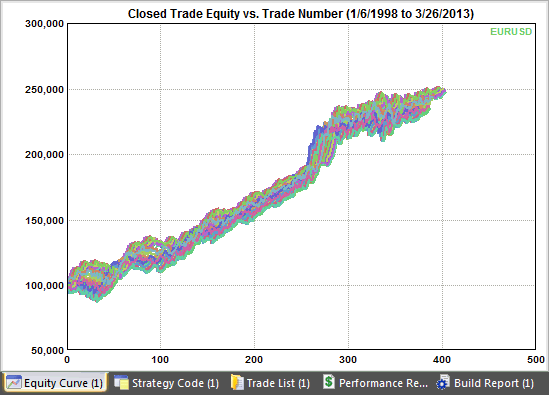Figure 5. Stress testing the forex strategy by varying the starting bar 99 times, for a total of 100 equity curves.

The Monte Carlo results from this test are shown in Table 3 below, where they're compared to the results for the original starting bar.

Table 3. Stress testing the forex strategy by varying the starting bar.

 Monte Carlo Results, 95% Results, Original Data Net Profit 130,168 147,855 Max Drawdown 19,033 19,033 Profit Factor 1.566 1.632 Percent Wins 59.2 59.4

It's also possible to modify everything together or to modify combinations of variables, such as modifying the strategy inputs at the same time as the price data. In Fig. 6, below, all three stress tests were performed together. This means the strategy inputs, price data, and starting bar were randomly modified at the same time prior to evaluating the strategy.Figure 6. Stress testing the forex strategy by varying the starting bar 99 times, for a total of 100 equity curves.

Clearly, this combination of stress tests is a severe test of the strategy's robustness. One or two of the equity curves shown in Fig. 6 appear to show a net negative (or nearly so) net profit. Only one equity curve approaches the original one. The Monte Carlo results based on this test are shown below in Table 4.

Table 4. Stress testing the forex strategy by varying the price data, strategy inputs, and starting bar.

 Monte Carlo Results, 95% Results, Original Data Net Profit 5,277 147,855 Max Drawdown 43,323 19,033 Profit Factor 1.017 1.632 Percent Wins 52.2 59.4

Summary and Conclusions

Over-fitting is always a concern when developing a trading strategy. So-called stress tests measure how robust a trading strategy is, which is an indication of whether or not the strategy is over-fit. While any variable that affects a trading strategy's results can potentially be the subject of a stress test, this article focused on three important factors in determining back-test results: the price data, the strategy's input values, and the starting bar for the back-test.

The strategy used to illustrate each stress test demonstrated moderate robustness with respect to the price data and input values and good robustness with respect to the starting bar. It's worthwhile to note that the example strategy had a three-year record of positive real-time tracking results, yet, in some cases, the stress test results were worse than the actual out-of-sample results achieved by the strategy. This suggests that the stress tests may have been too severe in those cases. This was particularly evident when all three tests were combined, as shown in Fig. 6 and Table 4.

The stress test for the strategy inputs may have been unrealistically strict in that it modified all the inputs for each test iteration. A better approach may be to apply the same method used to modify the price data, in which a price was modified with a specified probability. Rather than modifying all the inputs each time, a probability could be applied to determine if a given input should be modified. If so, it would be modified in the way described above; otherwise, the input would be unmodified.

It was shown how the stress test results could be analyzed using Monte Carlo analysis. This allowed us to quantify the results and provide an estimate of performance that was generally more conservative than the back-test results based on the original data.

The focus of the article was on testing a trading strategy after it had been developed. In principle, however, the same approach could be used as part of the strategy development process. In Adaptrade Builder, the strategies are evolved based on the back-tested performance on the in-sample period. Instead of using the performance obtained from back-testing the strategy on the original data, the Monte Carlo results at 95% confidence from the stress test could be used. The top strategies in the population would be the ones with the best Monte Carlo results, which would tend to drive the population towards robust strategies. Unfortunately, if each Monte Carlo analysis were based on N simulations, the build process would take N times as long using this approach.

Along with out-of-sample testing and other methods discussed in this series of articles, stress testing provides another tool to help identify robust trading strategies and avoid over-fitting. If applied as part of the strategy evaluation process, stress testing may help weed out strategies that are overly sensitive to changes in the trading environment, which could help avoid losses and increase your chances of success in the markets.

Mike Bryant

* All stress tests were performed using Adaptrade Builder.

HYPOTHETICAL OR SIMULATED PERFORMANCE RESULTS HAVE CERTAIN INHERENT LIMITATIONS. UNLIKE AN ACTUAL PERFORMANCE RECORD, SIMULATED RESULTS DO NOT REPRESENT ACTUAL TRADING. ALSO, SINCE THE TRADES HAVE NOT ACTUALLY BEEN EXECUTED, THE RESULTS MAY HAVE UNDER- OR OVER-COMPENSATED FOR THE IMPACT, IF ANY, OF CERTAIN MARKET FACTORS, SUCH AS LACK OF LIQUIDITY. SIMULATED TRADING PROGRAMS IN GENERAL ARE ALSO SUBJECT TO THE FACT THAT THEY ARE DESIGNED WITH THE BENEFIT OF HINDSIGHT. NO REPRESENTATION IS BEING MADE THAT ANY ACCOUNT WILL OR IS LIKELY TO ACHIEVE PROFITS OR LOSSES SIMILAR TO THOSE SHOWN.

If you'd like to be informed of new developments, news, and special offers from Adaptrade Software, please join our email list. Thank you.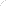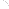Join Our Email List Email: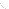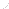For Email Marketing you can trust